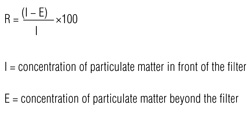# Calculations

This chapter explains various formulas which can be used to establish various aspects of the performance of your filters. The energy consumption, efficiency, permeability and filtration coefficient of filters are discussed in succession below.

## Energy consumption

By far the largest cost item in the area of filtration is energy consumption. Approx. 70 percent of the overall costs can be attributed to the energy consumption of your filter system. Calculating the energy consumption therefore provides clear insight into the total costs of operation. Energy consumption is expressed in kilowatt hours (kWh) which can be multiplied by the applicable energy rate to calculate the cost in euros. Important aspects in this regard are the volume of air passing through the air treatment cabinet, the average resistance, the operating hours and finally the efficiency of the fan. This final aspect generally varies from 0.6 to 0.7.## Efficiency of filters (filter efficiency)

Apart from the energy consumption, the efficiency of the filter is important. This calculation is used to establish the percentage of particulate matter trapped by the filter. Customers have the option of having this test performed at AFPRO Filters’ laboratory.## Permeability and penetration (%)

The penetration factor of a filter is another means of expressing its quality. It is calculated by dividing the concentration of particulate matter measured beyond the filter by that measured in front.